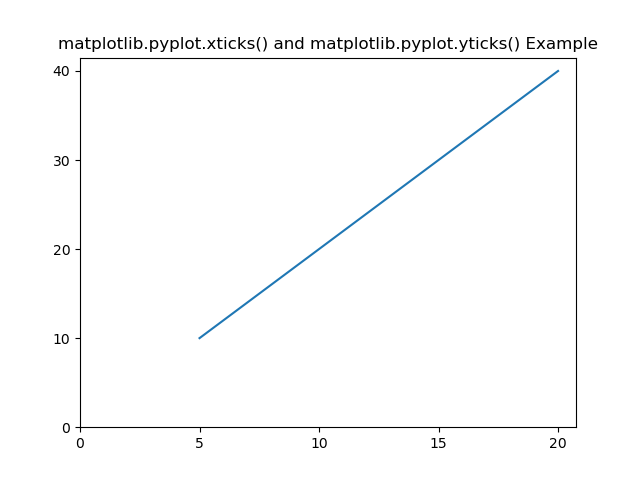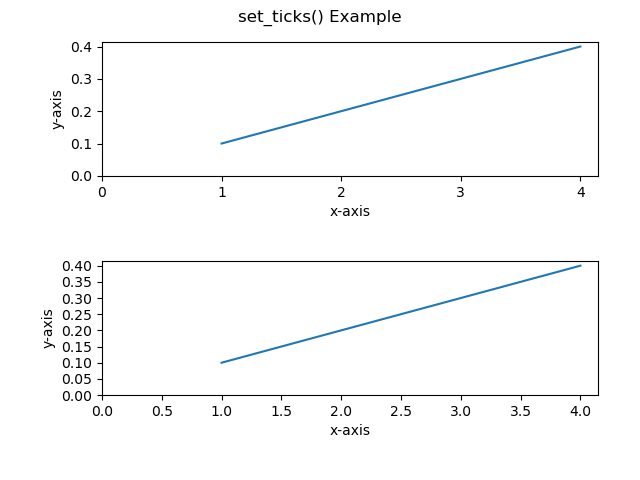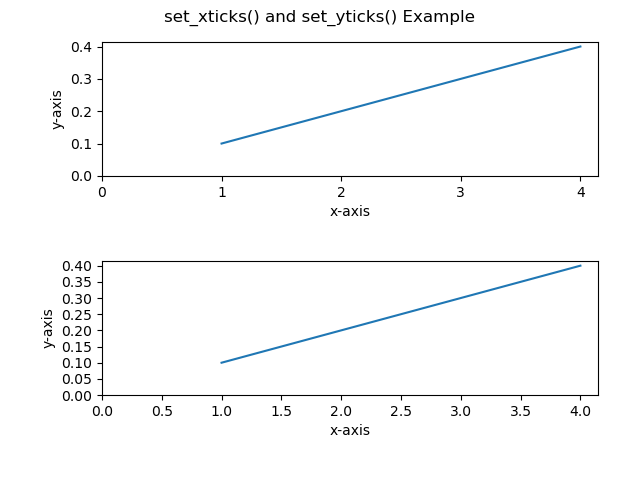Open in App
Not now

# Change the x or y ticks of a Matplotlib figure

• Difficulty Level : Basic
• Last Updated : 29 Oct, 2021

Matplotlib is a plotting library in Python to visualize data, inspired by MATLAB, meaning that the terms used (Axis, Figure, Plots) will be similar to those used in MATLAB. Pyplot is a module within the Matplotlib library which is a shell-like interface to Matplotlib module.

There are many ways to change the interval of ticks of axes of a plot of Matplotlib. Some of the easiest of them are discussed here.

### Method 1 : xticks() and yticks()

The xticks() and yticks() function takes a list object as an argument. The elements in the list denote the positions of the corresponding action where ticks will be displayed. We can also set labels of the ticks of the axes using these functions, but, here we will focus only on changing the interval of ticks of axes. To know more about these functions click on xticks() and yticks().

Syntax :

For x-axis : matplotlib.pyplot.xticks()

For y-axis : matplotlib.pyplot.yticks()

To create a list of ticks, we will use numpy.arange(start, stop, step) with start as the starting value for the ticks, stop as the non-inclusive ending value and step as the integer space between ticks.

Below example illustrate the matplotlib.pyplot.xticks() and matplotlib.pyplot.yticks() methods:

Example :

## Python3

 `#  Code to change the interval of ticks of axes using xticks() and yticks()` `# Importing libraries``import` `matplotlib.pyplot as plt``import` `numpy as np` `#Creating x-value and y-value of data``x ``=` `[``5``, ``10``, ``15``, ``20``]``y ``=` `[``10``, ``20``, ``30``, ``40``]` `# Plotting the data``plt.plot(x, y)` `# Setting the interval of ticks of x-axis to 5.``listOf_Xticks ``=` `np.arange(``0``, ``25``, ``5``)``plt.xticks(listOf_Xticks)` `# Setting the interval of ticks of y-axis to 10.``listOf_Yticks ``=` `np.arange(``0``, ``50``, ``10``)``plt.yticks(listOf_Yticks)` `# Giving title to the plot``plt.title(``'matplotlib.pyplot.xticks() and matplotlib.pyplot.yticks() Example'``)` `plt.show()`

Output :Here we can see that the range of ticks on x-axis is [0, 25) with an interval of 5 and on the y-axis is [0, 50) with an interval of 10. This is what we have set as the argument of np.arange().

Note: The above function (i.e. xticks() and yticks()) will not work for AxesSubplot object. For this, the below methods will work.

### Method 2 : set_ticks()

Similar to the above method, set_ticks() also takes a list object as an argument whose elements denote the position of ticks on the axis.

Syntax :

For x-axis :

AxesSubplot.xaxis.set_ticks()

For y-axis :

AxesSubplot.yaxis.set_ticks()

Note: This method will not work for matplotlib.pyplot object.

Below example illustrate the AxesSubplot.xaxis.set_ticks() and AxesSubplot.yaxis.set_ticks() methods:

Example :

## Python3

 `#  Code to change the interval of ticks of axes``# using set_ticks() method` `# Importing libraries``import` `matplotlib.pyplot as plt``import` `numpy as np``    ` `# Creating x-value and y-value of data``x ``=` `[``1``, ``2``, ``3``, ``4``]``y ``=` `[``0.1``, ``0.2``, ``0.3``, ``0.4``]``    ` `# Creating a subplot with 2 row and 1 column``fig, (axes1, axes2) ``=` `plt.subplots(``2``, ``1``)` `# Plotting first axes object i.e. axes1 and labeling``# its x and y axes``axes1.plot(x, y)``axes1.set_ylabel(``'y-axis'``)``axes1.set_xlabel(``'x-axis'``)` `# Setting the interval of ticks of x-axis to 1 and of y-axis``# to 0.1 of first axes i.e. axes1.``axes1.xaxis.set_ticks(np.arange(``0``, ``5``, ``1``))``axes1.yaxis.set_ticks(np.arange(``0``, ``0.5``, ``0.1``))` `# Plotting first axes object i.e. axes1 and labeling its``# x and y axes``axes2.plot(x, y)``axes2.set_ylabel(``'y-axis'``)``axes2.set_xlabel(``'x-axis'``)` `# Setting the interval of ticks of x-axis to 0.5 and``# of y-axis to 0.05 of second axes i.e. axes2.``axes2.xaxis.set_ticks(np.arange(``0``, ``4.5``, ``0.5``))``axes2.yaxis.set_ticks(np.arange(``0``, ``0.45``, ``0.05``))`  `# Giving title to the figure object i.e. fig``fig.suptitle(``'set_ticks() Example'``)``fig.tight_layout(pad``=``3.0``)` `plt.show()`

Output :### Method 3 : set_xticks() and set_yticks()

The set_xticks() and set_yticks() functions takes a list object as an argument. The elements in the list denote the positions of the corresponding action where ticks will be displayed.

Syntax :

For x-axis :

AxesSubplot.set_xticks()

For y-axis :

AxesSubplot.set_yticks()

Note: This method will not work for matplotlib.pyplot object.

Below example illustrate the AxesSubplot.set_xticks() and AxesSubplot.set_yticks() methods:

Example :

## Python3

 `#  Code to change the interval of ticks of axes``# using set_xticks() and set_yticks() methods` `# Importing libraries``import` `matplotlib.pyplot as plt``import` `numpy as np``    ` `#Creating x-value and y-value of data``x ``=` `[``1``, ``2``, ``3``, ``4``]``y ``=` `[``0.1``, ``0.2``, ``0.3``, ``0.4``]``    ` `# Creating a subplot with 2 row and 1 column``fig, (axes1, axes2) ``=` `plt.subplots(``2``, ``1``)` `# Plotting first axes object i.e. axes1 and``# labeling its x and y axes``axes1.plot(x, y)``axes1.set_ylabel(``'y-axis'``)``axes1.set_xlabel(``'x-axis'``)` `# Setting the interval of ticks of x-axis to 1 and of``# y-axis to 0.1 of first axes i.e. axes1.``axes1.set_xticks(np.arange(``0``, ``5``, ``1``))``axes1.set_yticks(np.arange(``0``, ``0.5``, ``0.1``))` `# Plotting first axes object i.e. axes1 and labeling``# its x and y axes``axes2.plot(x, y)``axes2.set_ylabel(``'y-axis'``)``axes2.set_xlabel(``'x-axis'``)` `# Setting the interval of ticks of x-axis to 0.5 and``# of y-axis to 0.05 of second axes i.e. axes2.``axes2.set_xticks(np.arange(``0``, ``4.5``, ``0.5``))``axes2.set_yticks(np.arange(``0``, ``0.45``, ``0.05``))`  `#Giving title to the figure object i.e. fig``fig.suptitle(``'set_xticks() and set_yticks() Example'``)``fig.tight_layout(pad``=``3.0``)` `plt.show()`

Output :My Personal Notes arrow_drop_up